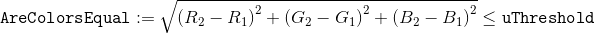﻿ Threshold Property (CompareBitmapCommand) | Leadtools.ImageProcessing.Core | Raster, Medical, Document Help
←Select platform
In This Topic ▼

# Threshold Property

Summary

An unsigned integer specifying the equality threshold for colors.

Syntax
C#
VB
C++
``public uint Threshold {get; set;} ``
````Public Property Threshold() As UInteger `
`   Get `
`   Set ````
````public:  `
`   property UInt32 Threshold `
`   { `
`      UInt32 get() `
`      void set(UInt32 value) `
`   } ````

#### Property Value

The maximum distance between two colors that can still be considered to be equal. The default value is 0.

Remarks

The Threshold defines the range in color values allowed and still be considered the same color. The distance is calculated in Euclidean RGB color space using the following equation:Example

Refer to the CompareBitmapCommand example.

Requirements